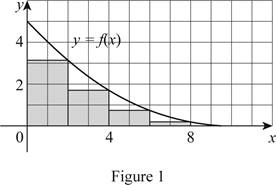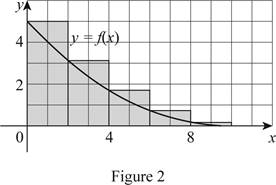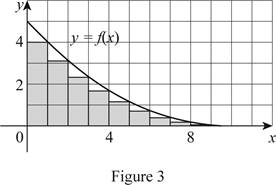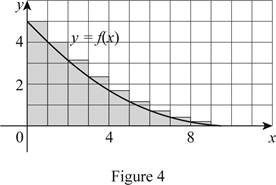# The lower estimate and upper estimate ofthe area under a graph using five rectangles.### Single Variable Calculus: Concepts...

4th Edition
James Stewart
Publisher: Cengage Learning
ISBN: 9781337687805### Single Variable Calculus: Concepts...

4th Edition
James Stewart
Publisher: Cengage Learning
ISBN: 9781337687805

#### Solutions

Chapter 5.1, Problem 1E

(a)

To determine

## The lower estimate and upper estimate ofthe area under a graph using five rectangles.

Expert Solution

The lower estimate of the area under the graph is 12.

The upper estimate of the area under the graph is 22.

### Explanation of Solution

Given information:

Take the curve as y=f(x).

Consider that the region lies between x=0 and x=10. Hence, the limits are a=0 and b=10.

Consider the number of rectangles as n=5.

Use the right end points of the curve as the lower estimate and the left end points of the curve as the upper estimate for decreasing behavior of the curve (f).

The expression to find the lower estimate of the areas of n rectangles (Rn) is shown below:

Rn=f(x1)Δx+f(x2)Δx+...+f(xn)Δx (1)

Here, the height of first rectangle is f(x1), the width is Δx, the height of the second rectangle is f(x2), and the height of nth rectangle is f(xn).

Draw five rectangles using right endpoints as shown in Figure (1).Find the width (Δx) using the relation:

Δx=ban (2)

Here, the upper limit is b, the lower limit is a, and the number of rectangles is n.

Substitute 10 for b, 0 for a and 5 for n in Equation (2).

Δx=1005=2

Refer to Figure (1),

Take the height of the first rectangle’s f(x1) value as 3.2, the height of the second rectangle’s f(x2) value as 1.8, the height of the third rectangle’s f(x3) value as 0.8, the height of the fourth rectangle’s f(x4) value as 0.2, and the height of the fifth rectangle’s f(x5) value as 0.

Substitute 5 for n, 3.2 for f(x1), 2 for Δx, 1.8 for f(x2), 0.8 for f(x3), 0.2 for f(x4), and 0 for f(x5) in Equation (1).

R5=(3.2×2)+(1.8×2)+(0.8×2)+(0.2×2)+(0×2)=6.4+3.6+1.6+0.4+0=12

Therefore, the lower estimate using right endpoints for n=5 is 12.

Draw five rectangles using left endpoints as shown in Figure (2).The expression to find the upper estimate of areas of n rectangles (Ln) is shown below:

Ln=f(x0)Δx+f(x1)Δx+...+f(xn1)Δx (3)

Here, the left endpoint height of the first rectangle is f(x0), the width is Δx,height of left endpoint of second rectangle is f(x1), and left endpoint height of nth rectangle is f(xn1).

Refer to Figure (2),

Take the height of the left endpoint f(x0) value as 5, the left endpoint height f(x1) value as 3.2, the left endpoint height f(x2) value as 1.8, the left endpoint height f(x3) value as 0.8, and the left endpoint height f(x4) value as 0.2.

Substitute 5 for n, 5 for f(x0), 2 for Δx, 3.2 for f(x1), 1.8 for f(x2), 0.8 for f(x3), and 0.2 for f(x4) in Equation (3).

L5=(5×2)+(3.2×2)+(1.8×2)+(0.8×2)+(0.2×2)=10+6.4+3.6+1.6+0.4=22

Therefore, the upper estimate using left endpoints for n=5 is 22.

(b)

To determine

### The lower estimate and upper estimate of the area using ten rectangles.

Expert Solution

The lower estimate of the area using ten rectangles is 14.4.

The upper estimate of the area using ten rectangles is 19.4.

### Explanation of Solution

Given information:

Take the curve as y=f(x).

Consider that the region lies between x=0 and x=10. So the limits are a=0 and b=10.

Consider the number of rectangles to be n=10.

Use the right end points of the curve as the lower estimate and the left end points of the curve as the upper estimate for the decreasing behavior of the curve (f).

Draw ten rectangles using right endpoints as shown in Figure (3).Substitute 10 for b, 0 for a and 10 for n in Equation (2).

Δx=ban=10010=1

Refer to Figure (3),

Take the height of the right endpoint f(x1) value as 4, the right endpoint height f(x2) value as 3.2, the right endpoint height f(x3) value as 2.5, the right endpoint height f(x4) value as 1.8, the right endpoint height f(x5) value as 1.3,the right endpoint height f(x6) value as 0.8, the right endpoint height f(x7) value as 0.5, the right endpoint height f(x8) value as 0.2, the right endpoint height f(x9) value as 0.1, and the right endpoint height f(x10) value as 0.

Substitute 10 for n, 4 for f(x1), 1 for Δx, 3.2 for f(x2), 2.5 for f(x3), 1.8 for f(x4), 1.3 for f(x5), 0.8 for f(x6), 0.5 for f(x7), 0.2 for f(x8), 0.1 for f(x9), and 0 for f(x10), in Equation (1).

R10=[f(x1)Δx+f(x2)Δx+f(x3)Δx+f(x4)Δx+f(x5)Δx+f(x6)Δx+f(x7)Δx+f(x8)Δx+f(x9)Δx+f(x10)Δx]=[(4×1)+(3.2×1)+(2.5×1)+(1.8×1)+(1.3×1)+(0.8×1)+(0.5×1)+(0.2×1)+(0.1×1)+(0×1)]=4+3.2+2.5+1.8+1.3+0.8+0.5+0.2+0.1+0=14.4

Therefore, the lower estimate using the right endpoints for n=10 is 14.4.

Draw ten rectangles using left endpoints as shown in Figure (4).The expression to find the upper estimate of the areas of the 10 rectangles (L10) is shown below:

L10=R10+f(x0)Δxf(x9)Δx (4)

Here, the lower estimate using the right endpoints for n=10 is R10, the height of the left uppermost rectangle is f(x0), and the height of the right lowermost is f(x9).

Refer to Figure (4),

Take the height of the left endpoint height f(x0) value as 5 and the left endpoint height f(x9) value as 0.

Substitute 14.4 for Rn, 5 for f(x0), 1 for Δx, and 0 for f(x9) in Equation (4).

L10=R10+f(x0)Δxf(x9)Δx=14.4+(5×1)(0×1)=14.4+50=19.4

Therefore, the upper estimate using the left endpoints for n=10 is 19.4.

### Have a homework question?

Subscribe to bartleby learn! Ask subject matter experts 30 homework questions each month. Plus, you’ll have access to millions of step-by-step textbook answers!